Quantum Numbers and Rules

# Quantum Numbers and Rules - Notes | Study Modern Physics for IIT JAM - Physics

 1 Crore+ students have signed up on EduRev. Have you?

Physical characteristics that are quantized—such as energy, charge, and angular momentum—are of such importance that names and symbols are given to them. The values of quantized entities are expressed in terms of quantum numbers, and the rules governing them are of the utmost importance in determining what nature is and does. This section covers some of the more important quantum numbers and rules—all of which apply in chemistry, material science, and far beyond the realm of atomic physics, where they were first discovered. Once again, we see how physics makes discoveries which enable other fields to grow.
The energy states of bound systems are quantized, because the particle wavelength can fit into the bounds of the system in only certain ways. This was elaborated for the hydrogen atom, for which the allowed energies are expressed as En ∝ 1/n, where n=1, 2, 3, .... We define
n to be the principal quantum number that labels the basic states of a system. The lowest-energy state has
n=1, the first excited state has
n=2, and so on. Thus the allowed values for the principal quantum number are
n=1, 2, 3, ....
This is more than just a numbering scheme, since the energy of the system, such as the hydrogen atom, can be expressed as some function of n, as can other characteristics (such as the orbital radii of the hydrogen atom).
The fact that the magnitude of angular momentum is quantized was first recognized by Bohr in relation to the hydrogen atom; it is now known to be true in general. With the development of quantum mechanics, it was found that the magnitude of angular momentum L can have only the values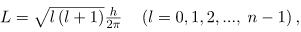Where l is defined to be the angular momentum quantum number. The rule for l in atoms is given in the parentheses. Given n, the value of l can be any integer from zero up to n-1. For example, if n=4, then l can be 0, 1, 2, or 3.
Note that for n=1, l can only be zero. This means that the ground-state angular momentum for hydrogen is actually zero, not
h/2π as Bohr proposed. The picture of circular orbits is not valid, because there would be angular momentum for any circular orbit. A more valid picture is the cloud of probability shown for the ground state of hydrogen in. The electron actually spends time in and near the nucleus. The reason the electron does not remain in the nucleus is related to Heisenberg’s uncertainty principle—the electron’s energy would have to be much too large to be confined to the small space of the nucleus. Now the first excited state of hydrogen has n=2, so that l can be either 0 or 1, according to the rule in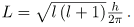Similarly, for n=3, l can be 0, 1, or 2. It is often most convenient to state the value of l, a simple integer, rather than calculating the value of L from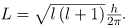For example, for l=2, we see that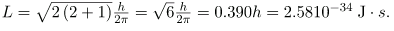It is much simpler to state l=2.
As recognized in the Zeeman effect, the direction of angular momentum is quantized. We now know this is true in all circumstances. It is found that the component of angular momentum along one direction in space, usually called the z-axis, can have only certain values of Lz. The direction in space must be related to something physical, such as the direction of the magnetic field at that location. This is an aspect of relativity. Direction has no meaning if there is nothing that varies with direction, as does magnetic force. The allowed values of Lz are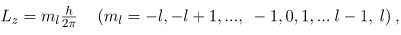Where Lz is the z-component of the angular momentum and ml is the angular momentum projection quantum number. The rule in parentheses for the values of ml is that it can range from -l to l in steps of one. For example, if l=2, then ml can have the five values –2, –1, 0, 1, and 2. Each ml corresponds to a different energy in the presence of a magnetic field, so that they are related to the splitting of spectral lines into discrete parts, as discussed in the preceding section. If the z-component of angular momentum can have only certain values, then the angular momentum can have only certain directions, as illustrated in.
The component of a given angular momentum along the z-axis (defined by the direction of a magnetic field) can have only certain values; these are shown here for l=1, for which ml=-1, 0, and +1. The direction of L is quantized in the sense that it can have only certain angles relative to the z-axis.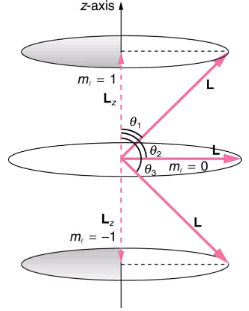Q.1 What Are the Allowed Directions?
Q.2 Calculate the angles that the angular momentum vector L can make with the z-axis for l=1, as illustrated in.
Strategy:
Represents the vectors L and Lz as usual, with arrows proportional to their magnitudes and pointing in the correct directions. L and Lz form a right triangle, with L being the hypotenuse and Lz the adjacent side. This means that the ratio of Lz to L is the cosine of the angle of interest. We can find L and Lz using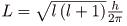and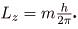Solution:
We are given l=1, so that ml can be +1, 0, or −1. Thus L has the value given by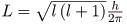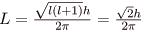Lz can have three values, given by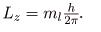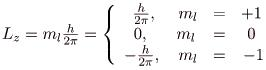As can be seen in,
cos θ Lz /L
and so for c= +1, we have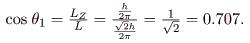Thus,  θ1 = cos-1 0.707 = 45.0.
Similarly, for m= 0, we find cos θ2 = 0; thus,
θ2 = 0; cos-1 0.707 = 90.0.
And for m= -1,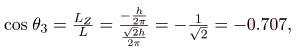so that θ3 = 0; cos-1 (-0.707) = 135.0.

Discussion:
The angles are consistent with the figure. Only the angle relative to the z-axis is quantized. L can point in any direction as long as it makes the proper angle with the z-axis. Thus the angular momentum vectors lie on cones as illustrated. This behavior is not observed on the large scale. To see how the correspondence principle holds here, consider that the smallest angle θin the example) is for the maximum value of m= 0, namely m= l. For that smallest angle,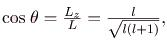which approaches 1 as l becomes very large. If cos θ = 1 then θ = 0. Furthermore, for large l, there are many values of ml, so that all angles become possible as l gets very large.

Intrinsic Spin Angular Momentum Is Quantized in Magnitude and Direction
There are two more quantum numbers of immediate concern. Both were first discovered for electrons in conjunction with fine structure in atomic spectra. It is now well established that electrons and other fundamental particles have intrinsic spin, roughly analogous to a planet spinning on its axis. This spin is a fundamental characteristic of particles, and only one magnitude of intrinsic spin is allowed for a given type of particle. Intrinsic angular momentum is quantized independently of orbital angular momentum. Additionally, the direction of the spin is also quantized. It has been found that the magnitude of the intrinsic (internal) spin angular momentum, S, of an electron is given b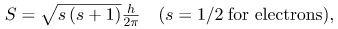where s is defined to be the spin quantum number. This is very similar to the quantization of L given in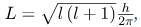except that the only value allowed for s for electrons is 1/2.
The direction of intrinsic spin is quantized, just as is the direction of orbital angular momentum. The direction of spin angular momentum along one direction in space, again called the z-axis, can have only the values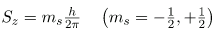for electrons. Sz is the z-component of spin angular momentum and ms is the spin projection quantum number. For electrons, s can only be 1/2, and ms  can be either +1/2 or –1/2. Spin projection ms + 1/2 is referred to as spin up, whereas ms = -1/2 is called spin down.

Intrinsic Spin
In later chapters, we will see that intrinsic spin is a characteristic of all subatomic particles. For some particles s is half-integral, whereas for others s is integral—there are crucial differences between half-integral spin particles and integral spin particles. Protons and neutrons, like electrons, have s=1/2, whereas photons have s=1, and other particles called pions have s=0, and so on.
To summarize, the state of a system, such as the precise nature of an electron in an atom, is determined by its particular quantum numbers. These are expressed in the form (n, l, ml, ms, the principal quantum number can have the values n=1, 2, 3, .... Once n is known, the values of the angular momentum quantum number are limited to l=1, 2, 3, ...,n-1. For a given value of l, the angular momentum projection quantum number can have only the values m=-l, +1, ...,-1, 0, 1, ..., l - 1, l. Electron spin is independent of n, l, and ml, always having s=1/2. The spin projection quantum number can have two values, m=1/2 or -1/2.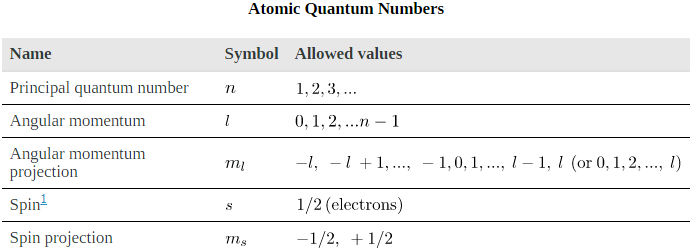several hydrogen states corresponding to different sets of quantum numbers. Note that these clouds of probability are the locations of electrons as determined by making repeated measurements—each measurement finds the electron in a definite location, with a greater chance of finding the electron in some places rather than others. With repeated measurements, the pattern of probability shown in the figure emerges. The clouds of probability do not look like nor do they correspond to classical orbits. The uncertainty principle actually prevents us and nature from knowing how the electron gets from one place to another, and so an orbit really does not exist as such. Nature on a small scale is again much different from that on the large scale.
Probability clouds for the electron in the ground state and several excited states of hydrogen. The nature of these states is determined by their sets of quantum numbers, here given as (n, l, ml . The ground state is (0, 0, 0); one of the possibilities for the second excited state is (3, 2, 1). The probability of finding the electron is indicated by the shade of color; the darker the coloring the greater the chance of finding the electron.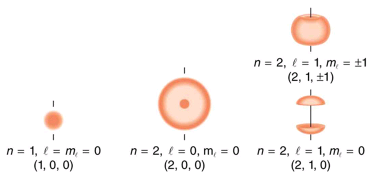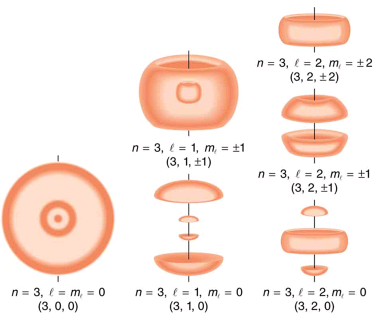We will see that the quantum numbers discussed in this section are valid for a broad range of particles and other systems, such as nuclei. Some quantum numbers, such as intrinsic spin, are related to fundamental classifications of subatomic particles, and they obey laws that will give us further insight into the substructure of matter and its interactions.

Section Summary:

• Quantum numbers are used to express the allowed values of quantized entities. The principal quantum number n labels the basic states of a system and is given by
n = 1, 2, 3,....
• The magnitude of angular momentum is given by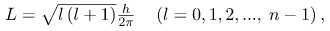where l is the angular momentum quantum number. The direction of angular momentum is quantized, in that its component along an axis defined by a magnetic field, called the z-axis is given by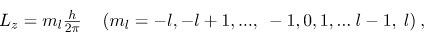where Lz is the z-component of the angular momentum and ml is the angular momentum projection quantum number. Similarly, the electron’s intrinsic spin angular momentum S is given by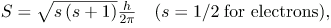s is defined to be the spin quantum number. Finally, the direction of the electron’s spin along the z-axis is given by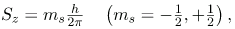where Sz is the z-component of spin angular momentum and ms is the spin projection quantum number. Spin projection m= + 1/2 is referred to as spin up, whereas ms = -1/2 is called spin down. Summarizes the atomic quantum numbers and their allowed values.
The document Quantum Numbers and Rules - Notes | Study Modern Physics for IIT JAM - Physics is a part of the Physics Course Modern Physics for IIT JAM.
All you need of Physics at this link: Physics

## Modern Physics for IIT JAM

54 videos|44 docs|15 tests
 Use Code STAYHOME200 and get INR 200 additional OFF

## Modern Physics for IIT JAM

54 videos|44 docs|15 tests

Track your progress, build streaks, highlight & save important lessons and more!

,

,

,

,

,

,

,

,

,

,

,

,

,

,

,

,

,

,

,

,

,

;